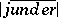# Priority method

A method used in recursion theory for the construction of sets with a simple structure (from the recursive point of view; in the simplest case recursively-enumerable sets), satisfying an infinite system of conditions of a definite kind. A feature of the permissible conditions is that for the satisfaction of a single condition of the system it is usually sufficient that in a simple set there is included a certain element, depending on the condition considered. However, in each construction stage (represented by a computational process in order to ensure the recursive simplicity of construction of the object looked for) every condition from the system (defining, in general, an infinite set of constructible objects) is represented by a finite approximation of it. The fact that the set to be constructed contains an element satisfying the approximation of the-th condition does not, however, ensure that this set will satisfy the-th condition itself. The priority method does allow one to circumvent this obstruction in certain cases. In doing this, the-th condition of the given system,is put in correspondence, during the construction process, with a natural number, which is a candidate for the role of the element whose inclusion in the set to be constructed allows one to satisfy the-th condition; this element is conveniently said to have been marked by(possibly endowed with additional indices). Every such candidate can be replaced, during the construction process (or, equivalently, its mark can be shifted); however, in the simplest priority constructions the sequence by which one tries to satisfy the given conditions is organized in such a way that each mark can change position only finitely many times, and in its final position the mark must necessarily be the mark of an element that guarantees that the respective condition is satisfied.